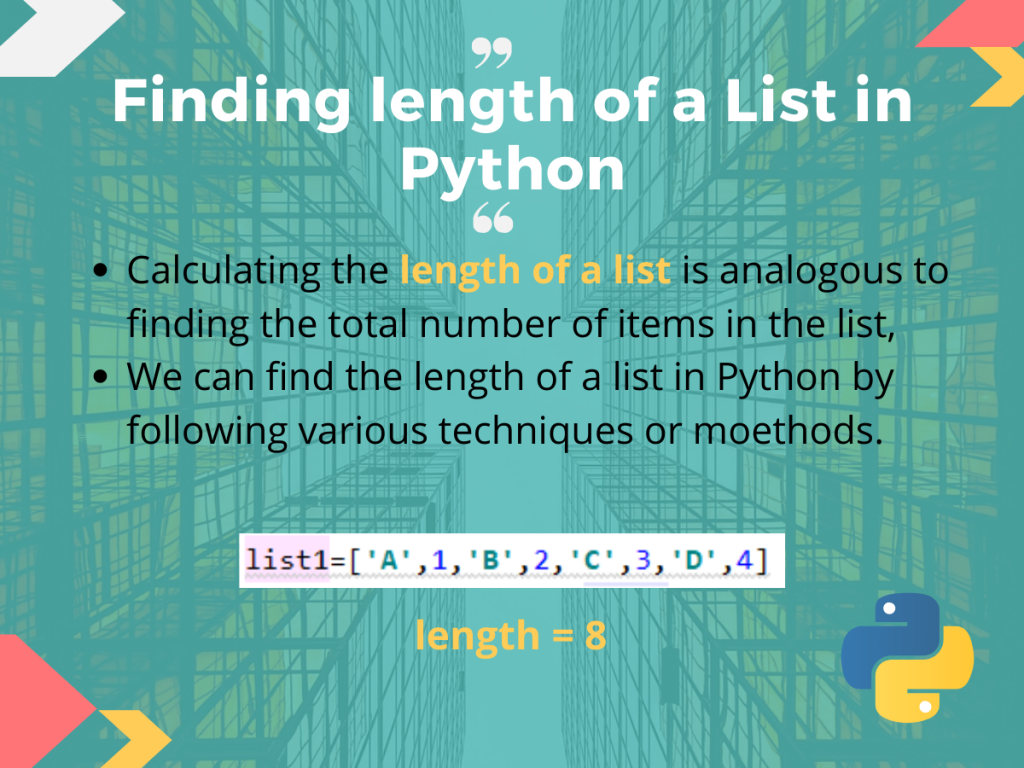# Length of a List in Python## Introduction

So, in this tutorial, we are going to discuss what we mean by the length of a list in Python, as well as how we can calculate it using various methods.

We know, Python List is a mutable as well as ordered sequence. It may contain heterogeneous items as well as homogenous ones. It is a vastly used data structure in Python. For traversal as well as for performing some other operations on a list, we sometimes need to find the length of the list.

## How to Calculate the length of a List?

Calculating the length or size of a list is analogous to finding the total number of items present in the same.

For example, if we have a list, list1:

```list1 = [5,4,7,2,9,6]
```

The length of the list `lis1` is 6. As the total number of elements or items is ‘6’.

## Methods of Finding the Length of a List in Python

The length of a list can be calculated in Python by applying various techniques. Below we are going to discuss them one-by-one in detail.

### 1. Using the len() method in Python

The built-in `len()` method in Python is widely used to calculate the length of any sequential data type. It calculates the number of elements or items in an object and returns the same as the length of the object.

So, we can directly get the length of a list, through passing the same into the built-in `len()` method. Let us see how.

```#given list
l=[11,22,33,44,55,66]

#printing the length using len()
print("The length of the list",l, "is :",len(l))
```

Output:

```The length of the list [11, 22, 33, 44, 55, 66] is : 6
```

In this code:

• We firstly take a list, `list1`
• Then we directly pass the list into the `len()` method and it returns the length of the list. This in our case is 6.

### 2. Custom Function to Calculate the Length of a List

Now let us define our own function in Python which will calculate the length of a list passed to it and return it where the function was called.

Below is the `calc_len()` function that we define to find the length of a list.

```def calc_len(l):
c=0
for i in l:
c=c+1
return c

#given list
list1=['A',1,'B',2,'C',3,'D',4]

#printing the length using calc_len()
print("The length of the list",list1, "is :",calc_len(list1))
```

Output:

• In the code above, we define a function `calc_len()` which takes the list whose length is to be found as a parameter
• Inside the `calc_len()` function, we initialize a counter c which is increased by 1 on each `for` loop iteration and stores the total number of items in the list, and finally return the counter c
• Hence, we pass the list, `list1` to our user-defined function
• `cal_len()` which returns us back the length of the list that we print directly.